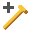# Support Overview

There are various ways of modelling support in SWedge:

• Individual bolts can be added to the model with the Add Bolt option.
• Shotcrete can be modelled with the Shotcrete option.
• An average support or loading pressure can be defined with the Pressure option.
• A force can be applied to the wedge with the External Force option.

It is important to remember that all of the methods of modelling support in SWedge, simply represent different ways of applying a force to the wedge. Like all other forces in the SWedge analysis, support forces are applied through the centroid of the wedge.

The different support options allow the user to choose whichever method is most convenient. For example:

## Bolts

• A bolt added with the Add Boltoption does not necessarily have to represent a single bolt but could represent the total support force applied by all bolts in a pattern, which have the same orientation. The magnitude of the bolt capacity can be entered as the total capacity of all bolts.

## External Force

• A support force can also simply be modelled as an External Force. An External Force is exactly equivalent to a bolt added with the Add Bolt option if the magnitude and orientation are the same, and the bolt model is ACTIVE. See the How Bolts are Implemented in SWedge topic for details.

## Pressure

• The Pressure option allows the user to specify a support or loading pressure, in terms of force per unit area of wedge face (either the slope face or the upper slope). This is the easiest way to model the effect of pattern bolting, or surcharge loads because the user does not have to calculate the total force or define individual bolts. When Pressure is applied, the total force applied to the wedge is automatically calculated by SWedge, based on the wedge face area(s).
• In a Probabilistic analysis, if the joint orientations are random variables, then the actual size and shape of the wedge will change with each simulation. In this case, the Pressure option is the recommended way to properly model support, since it will automatically account for the changing surface area of the wedge.

## Shotcrete

The passive support force applied by shotcrete can be modelled with the Shotcrete option. This will apply a force normal to the slope face, with a magnitude equal to the shear strength of the shotcrete, multiplied by the trace lengths of the joints on the slope face.\

## Bolt Properties

Bolt Propertiescan be defined from the toolbar or the Support menu. The properties are common to all bolts defined in the model. You can assign a particular pre-defined bolt property to any single bolt when you add/edit the bolt in the Add Spot Bolt Dialog.